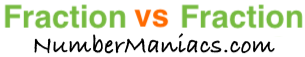1/2 vs 1/3People compare 1/2 vs 1/3 drywall, 1/2 vs 1/3 pipe, 1/2 vs 1/3 cup, 1/2 vs 1/3 ton, 1/2 vs 1/3 drill bit, 1/2 vs 1/3 poker, 1/2 vs 1/3 sump pump, 1/2 vs 1/3 garden hose, and many other items.

Is 1/2 greater than 1/3? Is 1/2 less than 1/3? What is the difference between 1/2 and 1/3? These and other questions are answered below so you can get a better perspective of 1/2 vs 1/3.

1/2 vs 1/3 Fractional Comparisons
1/2 is more than 1/3
The difference between 1/2 and 1/3 is 1/6
1/2 is 1/6 more than 1/3

1/2 vs 1/3 Decimal Comparisons
1/2 vs 1/3 as a decimal is 0.5 vs 0.333
The difference between 1/2 and 1/3 is 0.167
1/2 is 0.167 more than 1/3

1/2 vs 1/3 Percent Comparisons
1/2 vs 1/3 as a percent is 50% vs 33.3%
1/2 is 50 percent more than 1/3

Fraction vs Fraction
Submit another set of fractions that we can compare for you!

/
vs
/

The math behind the numbers
Calculations on this page are rounded to the nearest thousandth if necessary. Below is the math we used to analyze 1/2 vs 1/3:

To compare the size of 1/2 vs 1/3, we simply subtracted 1/3 from 1/2:

1/2 - 1/3 = |1/6|

To find 1/2 vs 1/3 as a decimal, we divided the numerator by the denominator for each fraction.

1 ÷ 2 = 0.5
1 ÷ 3 = 0.333

To find 1/2 vs 1/3 as a percent, we divided the numerator by the denominator and then multiplied the quotient by 100 for each fraction.

(1 ÷ 2) x 100 = 50%
(1 ÷ 3) x 100 = 33.3%

We found that 1/2 is 50 percent more than 1/3 by dividing the difference between the two fractions by the second fraction and then multiplied it by 100:

((1/6)/(1/3))×100 = 50%

That's it folks. We hope this page gave you a better understanding of 1/2 vs 1/3 with our explanations and conclusions.

1/2 vs 1/4
Here are the next two fractions we have compared and analyzed.